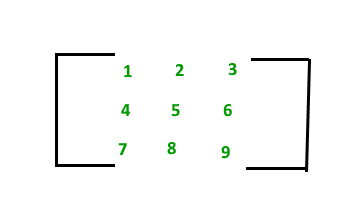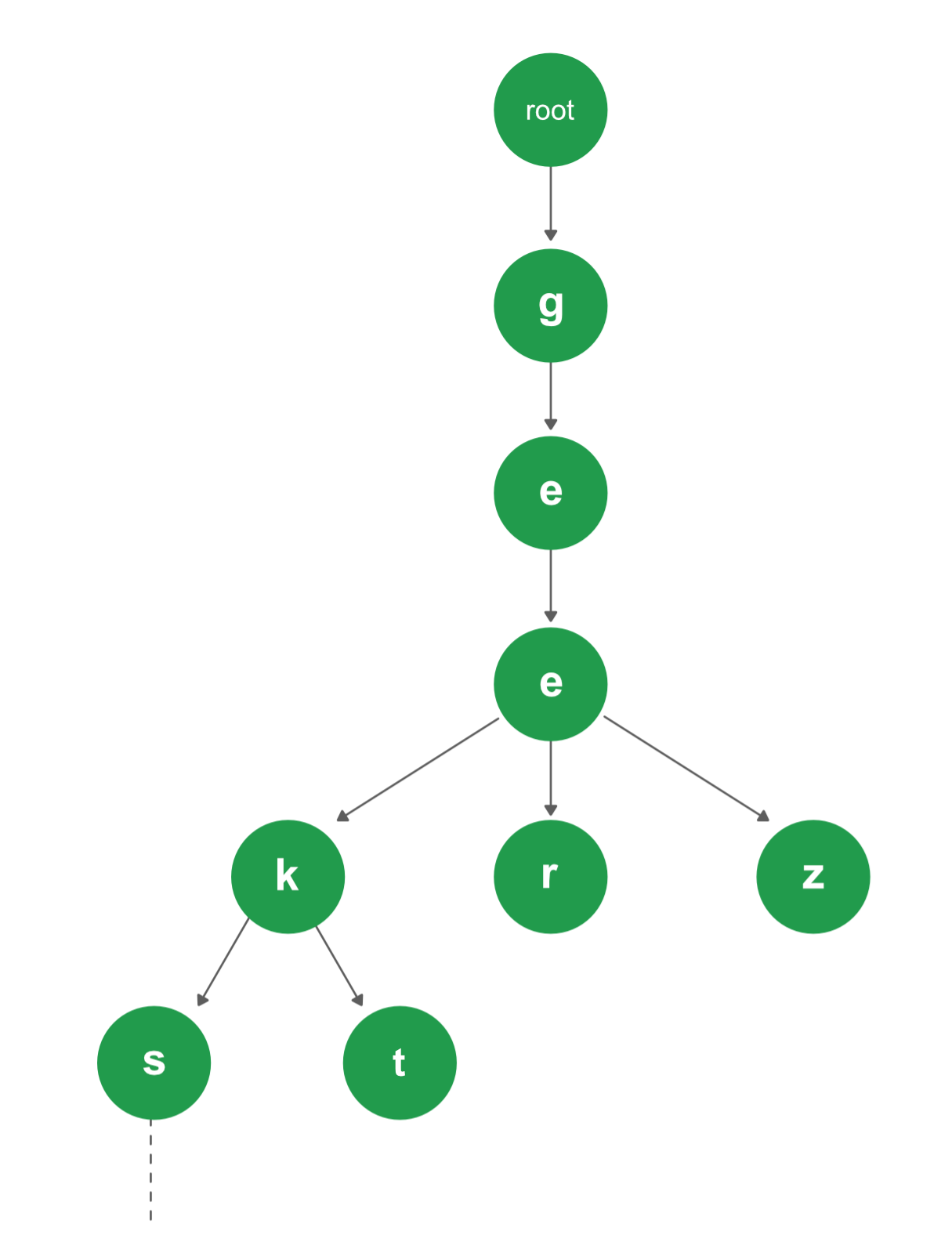Related Articles
Introduction to Data Structures | 10 most commonly used Data Structures
• Difficulty Level : Basic
• Last Updated : 18 Jul, 2019

A data structure is a particular way of organizing data in a computer so that it can be used effectively. The idea is to reduce the space and time complexities of different tasks.

Below is an overview of some popular data structures:

1. Array: An array is a collection of items stored at contiguous memory locations. The idea is to store multiple items of the same type together. This makes it easier to calculate the position of each element by simply adding an offset to a base value, i.e., the memory location of the first element of the array (generally denoted by the name of the array).2. Linked Lists: Like arrays, Linked List is a linear data structure. Unlike arrays, linked list elements are not stored at a contiguous location; the elements are linked using pointers.3. Stack: Stack is a linear data structure which follows a particular order in which the operations are performed. The order may be LIFO(Last In First Out) or FILO(First In Last Out).Mainly the following three basic operations are performed in the stack:

• Push: Adds an item in the stack. If the stack is full, then it is said to be an Overflow condition.
• Pop: Removes an item from the stack. The items are popped in the reversed order in which they are pushed. If the stack is empty, then it is said to be an Underflow condition.
• Peek or Top: Returns top element of the stack.
• isEmpty: Returns true if the stack is empty, else false.
4. Queue: Like Stack, Queue is a linear structure which follows a particular order in which the operations are performed. The order is First In First Out (FIFO). A good example of the queue is any queue of consumers for a resource where the consumer that came first is served first. The difference between stacks and queues is in removing. In a stack we remove the item the most recently added; in a queue, we remove the item the least recently added.Mainly the following four basic operations are performed on queue:

• Enqueue: Adds an item to the queue. If the queue is full, then it is said to be an Overflow condition.
• Dequeue: Removes an item from the queue. The items are popped in the same order in which they are pushed. If the queue is empty, then it is said to be an Underflow condition.
• Front: Get the front item from the queue.
• Rear: Get the last item from the queue.
5. Binary Tree: Unlike Arrays, Linked Lists, Stack and queues, which are linear data structures, trees are hierarchical data structures. A binary tree is a tree data structure in which each node has at most two children, which are referred to as the left child and the right child. It is implemented mainly using Links.

A Binary Tree is represented by a pointer to the topmost node in the tree. If the tree is empty, then the value of root is NULL. A Binary Tree node contains the following parts.

```1. Data
2. Pointer to left child
3. Pointer to the right child```
6. Binary Search Tree: In Binary Search Tree is a Binary Tree with the following additional properties:
• The left subtree of a node contains only nodes with keys less than the node’s key.
• The right subtree of a node contains only nodes with keys greater than the node’s key.
• The left and right subtree each must also be a binary search tree.
7. Heap: A Heap is a special Tree-based data structure in which the tree is a complete binary tree. Generally, Heaps can be of two types:
• Max-Heap: In a Max-Heap the key present at the root node must be greatest among the keys present at all of its children. The same property must be recursively true for all sub-trees in that Binary Tree.
• Min-Heap: In a Min-Heap the key present at the root node must be minimum among the keys present at all of its children. The same property must be recursively true for all sub-trees in that Binary Tree.8. Hashing Data Structure: Hashing is an important Data Structure which is designed to use a special function called the Hash function which is used to map a given value with a particular key for faster access of elements. The efficiency of mapping depends on the efficiency of the hash function used.

Let a hash function H(x) maps the value x at the index x%10 in an Array. For example, if the list of values is [11, 12, 13, 14, 15] it will be stored at positions {1, 2, 3, 4, 5} in the array or Hash table respectively.9. Matrix: A matrix represents a collection of numbers arranged in an order of rows and columns. It is necessary to enclose the elements of a matrix in parentheses or brackets.

A matrix with 9 elements is shown below.10. Trie: Trie is an efficient information reTrieval data structure. Using Trie, search complexities can be brought to an optimal limit (key length). If we store keys in the binary search tree, a well-balanced BST will need time proportional to M * log N, where M is maximum string length and N is the number of keys in the tree. Using Trie, we can search the key in O(M) time. However, the penalty is on Trie storage requirements.Attention reader! Don’t stop learning now. Get hold of all the important DSA concepts with the DSA Self Paced Course at a student-friendly price and become industry ready.  To complete your preparation from learning a language to DS Algo and many more,  please refer Complete Interview Preparation Course.

My Personal Notes arrow_drop_up